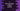# C program to find the remainder without using modulo operator### C program to find remainder without using modulo operator :

In this tutorial, we will learn how to find the remainder without using modulo operator (%) in C programming language. The algorithm that we are going to use is as below :

### Algorithm :

1. First read the number and divisor as entered by the user.
2. Keep subtracting the divisor from the number and set the value to the number till the number become less than divisor.
3. If the number becomes less than divisor, it should be the required remainder.
4. Print out the result.

For example, if the number is 10 and divisor is 3. First we will calculate 10 - 3 which is 7 and set this value to the number. Next time, it is 7 - 3 which is 4. Next number will be 4 - 3 = 1. Since 1 is less than 3, it is the remainder. Let’s take a look into the program :

### C program :

``````#include

int main()
{
//1
int no,divisor,remainder;

//2
printf("Enter the number : ");
scanf("%d",&no);

//3
printf("Enter the divisor : ");
scanf("%d",&divisor);

//4
while(no >= divisor){
no = no - divisor;
}

//5
remainder = no;

//6
printf("The remainder is %d ",remainder);

return 0;
}``````

### Explanation :

The commented numbers in the above program denotes the step number below :

1. Create three integer variables to store the value of the number (no), divisor (divisor) and remainder (remainder).
2. Ask the user to enter the variable and store it in variable no.
3. Ask the user to enter the divisor and store it in variable divisor.
4. Run one while loop. Check if no is greater than divisor and if yes, store the value of no - divisor in no. Do this till the value of no becomes less than divisor.
5. Assign the final value of no to the variable remainder.
6. Finally, print out the value of remainder.

### Sample Output :

``````Enter the number : 12
Enter the divisor : 4
The remainder is 0

Enter the number : 555
Enter the divisor : 4
The remainder is 3``````

### Where is the color and why codevscolor ?

Long story short, I love paintings and I paint on weekends. We(me and my wife) have one Youtube channel. Don't forget to subscribe ❤️❤️🤩